# C++中的引用

## 1 C++中的引用

### 1.1 引用的基本概念

• 引用可以看作是一个已定义变量的别名；
• 引用的语法：Type& name = var；
int a = 4;
int& b = a; // b是a的别名

b = 5;  // 操作b就是操作a


### 1.2 引用的意义

• 引用作为变量别名而存在，因此在一些场合可以替代指针。
• 引用相对于指针来说具有更好的可读性和实用性。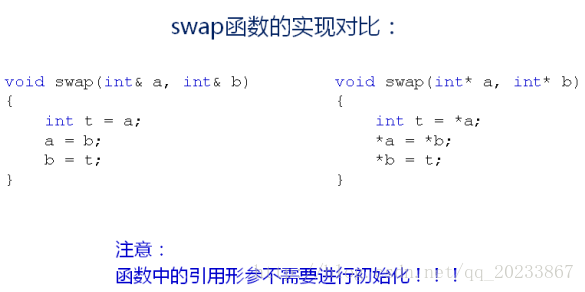### 1.3 const引用

const引用：

• 在C++中可以声明const引用；
• const Type& name = var；
• const引用让变量拥有只读属性（仅仅是不能通过该引用改变变量的值）。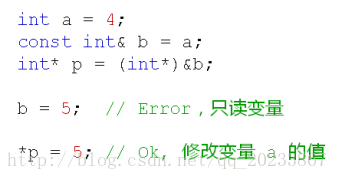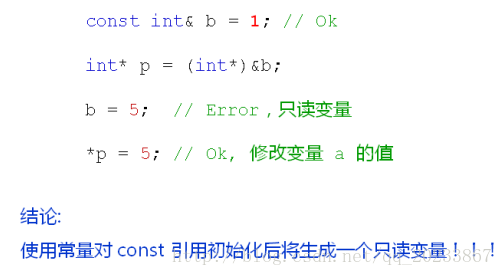#include <stdio.h>

void Example()
{
printf("Example:\n");

int a = 4;
const int& b = a;
int* p = (int*)&b;

//b = 5;

*p = 5;

printf("a = %d\n", a);
printf("b = %d\n", b);
}

void Demo()
{
printf("Demo:\n");

const int& c = 1;
int* p = (int*)&c;

//c = 5;

*p = 5;

printf("c = %d\n", c);
}

int main(int argc, char *argv[])
{
Example();

printf("\n");

Demo();

return 0;
}

/*
Example:
a = 5
b = 5

Demo:
c = 5

*/


#include <iostream>
#include <string>

using namespace std;

class Test1
{
public:
Test1()
{
cout << "Test1() " << this << endl;
}

~Test1()
{
cout << "~Test1() " << this << endl;
}
};

Test1 test()
{
Test1 t;

return t;
}

int main()
{
const Test1& t = test();

cout << "hello" << endl;

return 0;
}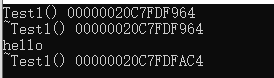#include <iostream>
#include <string>

using namespace std;

class Test1
{
public:
Test1()
{
cout << "Test1() " << this << endl;
}

~Test1()
{
cout << "~Test1() " << this << endl;
}
};

Test1 test()
{
Test1 t;

return t;
}

int main()
{
test();

cout << "hello" << endl;

return 0;
}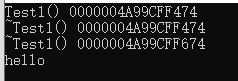## 2 引用的本质

### 2.1 引用占用的空间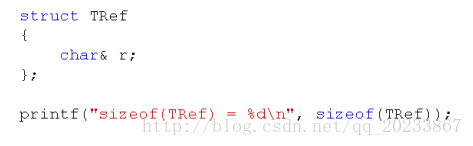#include <stdio.h>

struct TRef
{
char& r;
};

int main(int argc, char *argv[])
{
char c = 'c';
char& rc = c;
TRef ref = { c };

printf("sizeof(char&) = %d\n", sizeof(char&));  //1
printf("sizeof(rc) = %d\n", sizeof(rc));    //sizeof(c) = 1

printf("sizeof(TRef) = %d\n", sizeof(TRef));    //4
printf("sizeof(ref.r) = %d\n", sizeof(ref.r));  //1

return 0;
}



### 2.2 引用的本质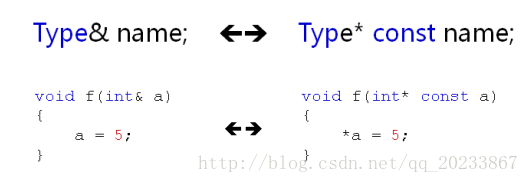1. C++编译器在编译过程中使用常量指针作为引用的内部实现，因此引用所占用的空间大小与指针相同。
2. 从使用的角度，引用只是一个别名，C++为了实用性而隐藏了引用的存储空间这一细节。
#include <stdio.h>

struct TRef
{
char* before;
char& ref;
char* after;
};

int main(int argc, char* argv[])
{
char a = 'a';
char& b = a;

/*
char& b = a; 对应的汇编语言如下（举例）：

lea eax, [a]
mov dword ptr [b], eax
*/
char c = 'c';

TRef r = {&a, b, &c};

printf("sizeof(r) = %d\n", sizeof(r));//12
printf("sizeof(r.before) = %d\n", sizeof(r.before));//4
printf("sizeof(r.after) = %d\n", sizeof(r.after));//4
printf("&r.before = %p\n", &r.before);//0xbf8a300c
printf("&r.after = %p\n", &r.after);//0xbf8a3014

return 0;
}



• 功能性：可以满足多数需要使用指针的场合。
• 安全性：可以避开由于指针操作不当而带来的内存错误。
• 操作性：简单易用，又不失功能强大。

## 3 引用和指针的关系

• 值为一个内存地址，不需要初始化，可以保存不同的地址；
• 通过指针可以访问对应内存地址中的值；
• 指针可以被const修饰成为常量或者只读变量。

• 对引用的操作（赋值、取地址等）都会传递到代表的变量上；
• cosnt引用使其代表的变量具有只读属性；
• 引用必须在定义时初始化，之后无法代表其它变量。

• 引用与指针没有任何关系；
• 引用是变量的新名字，操作引用就是操作对应的变量。

• 为了支持新概念“引用”必须要有一个有效的解决方案；
• 在编译器内部，使用常量指针来实现“引用”；
• 因此“引用”在定义时必须初始化。

• 当进行C++编程时，直接站在使用者的角度看待引用，与指针毫无关系，引用就是变量的别名；
• 当对C++代码进行调试分析时，一些特殊情况，可以考虑站在C++编译器的角度看待引用。

int a = 1;
int b = 2;
int *pc = new int(3);
int& array[] = {a, b, *pc};

// 注：C++中不支持引用数组。



#include <stdio.h>

int a = 1;

struct SV
{
int& x;
int& y;
int& z;
};

int main()
{
int b = 2;
int* pc = new int(3);
SV sv = {a, b, *pc};
int& array[] = {a, b, *pc}; // &array - &array = ?  Expected ==> 4 error!!!

printf("&sv.x = %p\n", &sv.x);
printf("&sv.y = %p\n", &sv.y);
printf("&sv.z = %p\n", &sv.z);

delete pc;

return 0;
}



1. C++深度解析教程
07-098157
02-0332
04-27157
05-27727
03-13256
03-26317
02-274406
01-03361
11-28201
12-14592
12-281724
01-14383
01-1048
12-223383
07-22813
02-06367

### “相关推荐”对你有帮助么？

•非常没帮助
•没帮助
•一般
•有帮助
•非常有帮助被折叠的  条评论 为什么被折叠?到【灌水乐园】发言SlowIsFastLemon

¥2 ¥4 ¥6 ¥10 ¥20余额支付 (余额：-- )扫码支付获取中扫码支付点击重新获取扫码支付1.余额是钱包充值的虚拟货币，按照1:1的比例进行支付金额的抵扣。
2.余额无法直接购买下载，可以购买VIP、C币套餐、付费专栏及课程。余额充值